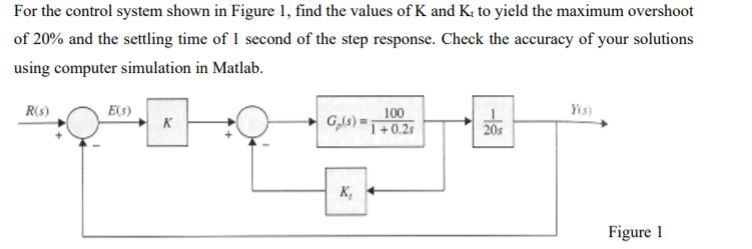# Question For the control system shown in Figure 1, find the values of K and Kto yield the maximum overshoot of 20% and the settling time of 1 second of the step response. Check the accuracy of your solutions using computer simulation in Matlab. Figure 1LMQ6L0 The Asker · Electrical EngineeringTranscribed Image Text: For the control system shown in Figure 1, find the values of K and Kto yield the maximum overshoot of 20% and the settling time of 1 second of the step response. Check the accuracy of your solutions using computer simulation in Matlab. Figure 1
More
Transcribed Image Text: For the control system shown in Figure 1, find the values of K and Kto yield the maximum overshoot of 20% and the settling time of 1 second of the step response. Check the accuracy of your solutions using computer simulation in Matlab. Figure 1